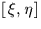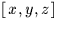Next: SLA_SEP - Angle Between 2 Points on Sphere
Up: SUBPROGRAM SPECIFICATIONS
Previous: SLA_RVLSRK - RV Corrn to Kinematical LSR

## SLA_S2TP - Spherical to Tangent Plane

ACTION:
Projection of spherical coordinates onto the tangent plane (single precision).

CALL:
CALL sla_S2TP (RA, DEC, RAZ, DECZ, XI, ETA, J)

GIVEN:

 R RA,DEC spherical coordinates of star (radians) RAZ,DECZ R spherical coordinates of tangent point (radians)

RETURNED:

 R XI,ETA tangent plane coordinates (radians) J I status: 0 = OK, star on tangent plane 1 = error, star too far from axis 2 = error, antistar on tangent plane 3 = error, antistar too far from axis

NOTES:
1.
The projection is called the gnomonic projection; the Cartesian coordinatesare called standard coordinates. The latter are in units of the distance from the tangent plane to the projection point, i.e. radians near the origin.
2.
When working inrather than spherical coordinates, the equivalent Cartesian routine sla_V2TP is available.Next: SLA_SEP - Angle Between 2 Points on Sphere
Up: SUBPROGRAM SPECIFICATIONS
Previous: SLA_RVLSRK - RV Corrn to Kinematical LSR

SLALIB --- Positional Astronomy Library